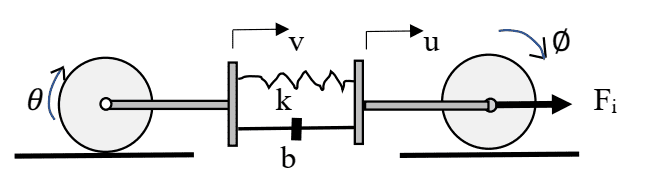# Dynamics Problem with a Driving Force, 2 Wheels, a Spring and a Damper

• Engineering
• rakan

#### rakan

Homework Statement
The input to the system shown below is the force F_i(t). The two identical wheels with
radius r roll without slip. Each wheel has mass M_w and rotational inertia 𝐽 = 𝑀_𝑤 𝑟^2/2; the connector
bars each have mass M_b. The spring is linear with stiffness K and the viscous damper is linear with coefficient b. The bearings at the center of the wheels are frictionless.

4.1 Draw the free body diagram for getting the component equations for the system shown below.

4.2 Write the component equations.

4.3 Verify that the component equations combine and simplify to the following simultaneous
equations:
(1.5*M_w + M_𝑏)𝒗̈ + 𝒃𝒗̇ + 𝒌𝒗 = 𝒃𝒖̇ + 𝒌𝒖
(1.5*M_w + M_𝑏)𝒖̈ + 𝒃𝒖̇ + 𝒌𝒖 = 𝒃𝒗̇ + 𝒌𝒗 + 𝑭_𝒊

4.4 Explain why four state variables are required to simulate this system using ode45; what are
these state variables and what are the equations for their derivatives?
𝒙̇_𝟏 =? 𝒙̇_𝟐 =? 𝒙̇_𝟑 =? 𝒙̇_𝟒 =?
Relevant Equations
(1.5*M_w + M_𝑏)𝒗̈ + 𝒃𝒗̇ + 𝒌𝒗 = 𝒃𝒖̇ + 𝒌𝒖
(1.5*M_w + M_𝑏)𝒖̈ + 𝒃𝒖̇ + 𝒌𝒖 = 𝒃𝒗̇ + 𝒌𝒗 + 𝑭_𝒊What are your thoughts so far?